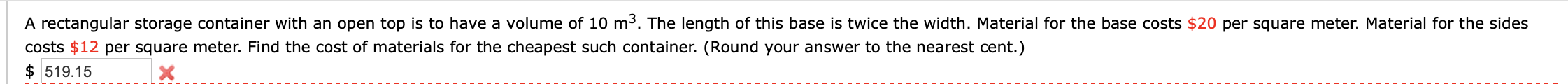# A rectangular storage container with an open top is to have a volume of 10 m3. The length of this base is twice the width. Material for the base costs \$20 per square meter. Material for the sidescosts \$12 per square meter. Find the cost of materials for the cheapest such container. (Round your answer to the nearest cent.)\$ 519.15

Question
1 viewshelp_outlineImage TranscriptioncloseA rectangular storage container with an open top is to have a volume of 10 m3. The length of this base is twice the width. Material for the base costs \$20 per square meter. Material for the sides costs \$12 per square meter. Find the cost of materials for the cheapest such container. (Round your answer to the nearest cent.) \$ 519.15 fullscreen
check_circle

star
star
star
star
star
1 Rating
Step 1

Let L, W & H be the length, width and height.

• L = 2W
• Volume, V = L x W x H = 2W x W x H = 2W2H = 10
• Hence, W2H = 5. Hence, H = 5 / W2
• Area of the base = L x W = 2W x W = 2W2
• Area of the four sides = 2 x (L x H + W x H) = 2 x (2W x H + W x H) = 6WH
• Material for the base costs \$20 per square meter. Material for the sides costs \$12 per square meter.
• The cost of materials = C = 20 x 2W+ 12 x 6WH = 40W+ 72WH = 40W+ 72W x (5 / W2) = 40W+ 360 / W
Step 2

In order to minimze the cost,

• its first derivative with resepct to W should be zero.
• And it's double derivative with respect to W should be positive.
Step 3

(Recall the famous rule of differentiation: d(xn) / dx = nxn-1)

dC/dW = 80W - 360 / W2 = 0

Hence, W3&nbs...

### Want to see the full answer?

See Solution

#### Want to see this answer and more?

Solutions are written by subject experts who are available 24/7. Questions are typically answered within 1 hour.*

See Solution
*Response times may vary by subject and question.
Tagged in

### Derivative